Courses

# Basics of Series Mathematics Notes | EduRev

## Mathematics : Basics of Series Mathematics Notes | EduRev

The document Basics of Series Mathematics Notes | EduRev is a part of the Mathematics Course Algebra for IIT JAM Mathematics.
All you need of Mathematics at this link: Mathematics

In this section we will introduce the topic that we will be discussing for the rest of this chapter. That topic is infinite series. So just what is an infinite series? Well, let’s start with a sequence {an}n=1 (note the n=1 is for convenience, it can be anything) and define the following,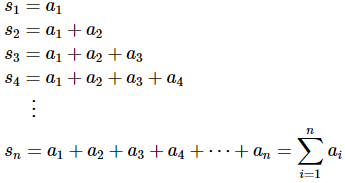The sn are called partial sums and notice that they will form a sequence, {sn}n=1. Also recall that the Σ is used to represent this summation and called a variety of names. The most common names are : series notation, summation notation, and sigma notation.
You should have seen this notation, at least briefly, back when you saw the definition of a definite integral in Calculus I. If you need a quick refresher on summation notation see the review of summation notation in the Calculus I notes.
Now back to series. We want to take a look at the limit of the sequence of partial sums,{sn}n=1. To make the notation go a little easier we’ll define,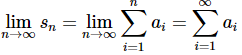We will call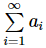ai  an infinite series and note that the series “starts” at i=1 because that is where our original sequence, {an}n=1, started. Had our original sequence started at 2 then our infinite series would also have started at 2. The infinite series will start at the same value that the sequence of terms (as opposed to the sequence of partial sums) starts.
It is important to note that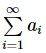is really nothing more than a convenient notation for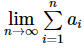so we do not need to keep writing the limit down. We do, however, always need to remind ourselves that we really do have a limit there!
If the sequence of partial sums, {sn}n=1, is convergent and its limit is finite then we also call the infinite series,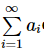convergent and if the sequence of partial sums is divergent then the infinite series is also called divergent.
Note that sometimes it is convenient to write the infinite series as,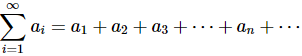We do have to be careful with this however. This implies that an infinite series is just an infinite sum of terms and as we’ll see in the next section this is not really true for many series.
In the next section we’re going to be discussing in greater detail the value of an infinite series, provided it has one of course as well as the ideas of convergence and divergence.
This section is going to be devoted mostly to notational issues as well as making sure we can do some basic manipulations with infinite series so we are ready for them when we need to be able to deal with them in later sections.
First, we should note that in most of this chapter we will refer to infinite series as simply series. If we ever need to work with both infinite and finite series we’ll be more careful with terminology, but in most sections we’ll be dealing exclusively with infinite series and so we’ll just call them series.
Now, in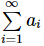the i is called the index of summation or just index for short and note that the letter we use to represent the index does not matter. So for example the following series are all the same. The only difference is the letter we’ve used for the index.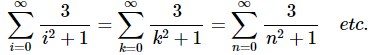It is important to again note that the index will start at whatever value the sequence of series terms starts at and this can literally be anything. So far we’ve used n=0 and n=1 but the index could have started anywhere. In fact, we will usually use ∑an to represent an infinite series in which the starting point for the index is not important. When we drop the initial value of the index we’ll also drop the infinity from the top so don’t forget that it is still technically there.
We will be dropping the initial value of the index in quite a few facts and theorems that we’ll be seeing throughout this chapter. In these facts/theorems the starting point of the series will not affect the result and so to simplify the notation and to avoid giving the impression that the starting point is important we will drop the index from the notation. Do not forget however, that there is a starting point and that this will be an infinite series.
Note however, that if we do put an initial value of the index on a series in a fact/theorem it is there because it really does need to be there.
Now that some of the notational issues are out of the way we need to start thinking about various ways that we can manipulate series.
We’ll start this off with basic arithmetic with infinite series as we’ll need to be able to do that on occasion. We have the following properties.
Properties
If ∑an and ∑bn are both convergent series then,
1. ∑can, where c is any number, is also convergent and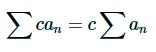2.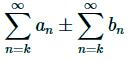is also convergent and,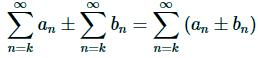The first property is simply telling us that we can always factor a multiplicative constant out of an infinite series and again recall that if we don’t put in an initial value of the index that the series can start at any value. Also recall that in these cases we won’t put an infinity at the top either.
The second property says that if we add/subtract series all we really need to do is add/subtract the series terms. Note as well that in order to add/subtract series we need to make sure that both have the same initial value of the index and the new series will also start at this value.
Before we move on to a different topic let’s discuss multiplication of series briefly. We’ll start both series at n=0 for a later formula and then note that,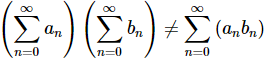To convince yourself that this isn’t true consider the following product of two finite sums.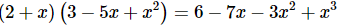Yeah, it was just the multiplication of two polynomials. Each is a finite sum and so it makes the point. In doing the multiplication we didn’t just multiply the constant terms, then the x terms, etc. Instead we had to distribute the 2 through the second polynomial, then distribute the x through the second polynomial and finally combine like terms.
Multiplying infinite series (even though we said we can’t think of an infinite series as an infinite sum) needs to be done in the same manner. With multiplication we’re really asking us to do the following,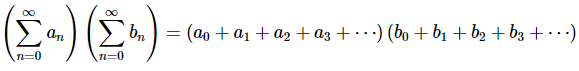To do this multiplication we would have to distribute the a0 through the second term, distribute the a1 through, etc then combine like terms. This is pretty much impossible since both series have an infinite set of terms in them, however the following formula can be used to determine the product of two series.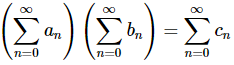where,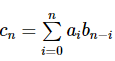We also can’t say a lot about the convergence of the product. Even if both of the original series are convergent it is possible for the product to be divergent. The reality is that multiplication of series is a somewhat difficult process and in general is avoided if possible. We will take a brief look at it towards the end of the chapter when we’ve got more work under our belt and we run across a situation where it might actually be what we want to do. Until then, don’t worry about multiplying series.
The next topic that we need to discuss in this section is that of index shift. To be honest this is not a topic that we’ll see all that often in this course. In fact, we’ll use it once in the next section and then not use it again in all likelihood. Despite the fact that we won’t use it much in this course doesn’t mean however that it isn’t used often in other classes where you might run across series. So, we will cover it briefly here so that you can say you’ve seen it.
The basic idea behind index shifts is to start a series at a different value for whatever the reason (and yes, there are legitimate reasons for doing that).
Consider the following series,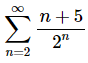Suppose that for some reason we wanted to start this series at n=0, but we didn’t want to change the value of the series. This means that we can’t just change the n=2 to n=0 as this would add in two new terms to the series and thus change its value.
Performing an index shift is a fairly simple process to do. We’ll start by defining a new index, say i, as follows,
i=n−2
Now, when n=2, we will get i=0. Notice as well that if n=∞ then i=∞−2=∞, so only the lower limit will change here. Next, we can solve this for n to get,
n=i+2
We can now completely rewrite the series in terms of the index i instead of the index n simply by plugging in our equation for n in terms of i.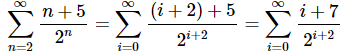To finish the problem out we’ll recall that the letter we used for the index doesn’t matter and so we’ll change the final i back into an n to get,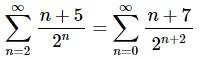To convince yourselves that these really are the same summation let’s write out the first couple of terms for each of them,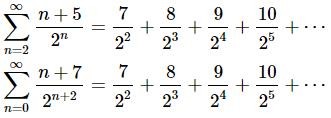So, sure enough the two series do have exactly the same terms.
There is actually an easier way to do an index shift. The method given above is the technically correct way of doing an index shift. However, notice in the above example we decreased the initial value of the index by 2 and all the n’s in the series terms increased by 2 as well.
This will always work in this manner. If we decrease the initial value of the index by a set amount then all the other n’s in the series term will increase by the same amount.Likewise, if we increase the initial value of the index by a set amount, then all the n’s in the series term will decrease by the same amount.
Let’s do a couple of examples using this shorthand method for doing index shifts.

Example 1: Perform the following index shifts.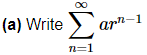as a series that starts at n=0.
In this case we need to decrease the initial value by 1 and so the n’s (okay the single n) in the term must increase by 1 as well.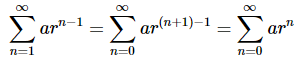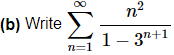as a series that starts at n=3.
For this problem we want to increase the initial value by 2 and so all the n’s in the series term must decrease by 2.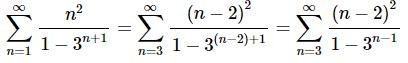The final topic in this section is again a topic that we’ll not be seeing all that often in this class, although we will be seeing it more often than the index shifts. This final topic is really more about alternate ways to write series when the situation requires it.
Let’s start with the following series and note that the n=1 starting point is only for convenience since we need to start the series somewhere.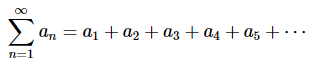Notice that if we ignore the first term the remaining terms will also be a series that will start at n=2 instead of n=1 So, we can rewrite the original series as follows,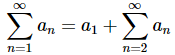In this example we say that we’ve stripped out the first term.
We could have stripped out more terms if we wanted to. In the following series we’ve stripped out the first two terms and the first four terms respectively.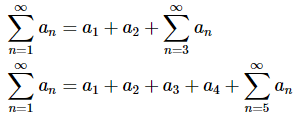Being able to strip out terms will, on occasion, simplify our work or allow us to reuse a prior result so it’s an important idea to remember.
Notice that in the second example above we could have also denoted the four terms that we stripped out as a finite series as follows,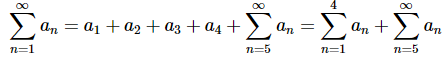This is a convenient notation when we are stripping out a large number of terms or if we need to strip out an undetermined number of terms. In general, we can write a series as follows,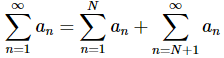We’ll leave this section with an important warning about terminology. Don’t get sequences and series confused! A sequence is a list of numbers written in a specific order while an infinite series is a limit of a sequence of finite series and hence, if it exists will be a single value.
So, once again, a sequence is a list of numbers while a series is a single number, provided it makes sense to even compute the series. Students will often confuse the two and try to use facts pertaining to one on the other. However, since they are different beasts this just won’t work. There will be problems where we are using both sequences and series so we’ll always have to remember that they are different.

Offer running on EduRev: Apply code STAYHOME200 to get INR 200 off on our premium plan EduRev Infinity!

58 docs

,

,

,

,

,

,

,

,

,

,

,

,

,

,

,

,

,

,

,

,

,

;